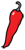The Grand Locus / Life for statistical sciences

the Blog

## A tutorial on t-SNE (2)In this post I explain what perplexity is and how it is used to parametrize t-SNE. This post is the second part of a tutorial on t-SNE. The first part introduces dimensionality reduction and presents the main ideas of t-SNE. This is where you should start if you are not already familiar with t-SNE.

### What is perplexity?

Before you read on, pick a number at random between 1 and 10 and ask yourself whether I can guess it. It looks like my chances are 1 in 10 so you may think “no there is no way”. In fact, there is a 28% chance that you chose the number 7, so my chances of guessing are higher than you may have thought initially. In this situation, the random variable is somewhat predictable but not completely. How could we quantify that?

To answer the questions, let us count the possible samples from this distribution. We ask $N$ people to choose a number at random between 1 and 10 and we record their answers $(x_1, x_2, \ldots, x_N)$. The number 1 shows up with probability $p_1 = 0.034$ so the total in the sample is approximately \$(n_1...